## How to Manipulate a Faust

This livescript is intended to gently introduce the operations available to manipulate a Faust object. It comes after the first livescript (available here for download or here as a web page), so it's assumed you already know how to create a Faust object from one way or another.
Keep in mind that a full API doc is available here every time you need details. In particular the Faust class is documented here.
NOTE: the livescript is made to be executed sequentially, otherwise, skipping some cells, you would end up on an import error.

1. Getting Basic Information about a Faust Object
1.1 Obtaining Dimension and Scalar Type Information
1.2 Obtaining Other Faust Specific Information
1.3 Plotting a Faust
2. Faust Algebra and other Operations
2.1 Transpose, conjugate, transconjugate
2.3 Faust Multiplication by a Vector or a Matrix
2.4 Faust Norms
2.5 Faust Normalizations
2.6 Faust Concatenation
2.7 Faust Indexing and Slicing

### 1. Getting Basic Information about a Faust Object

First of all, given any object, you might ask yourself if it's a Faust or not (typically when you receive an object in a function, matlab being built on dynamic types, you can't say for sure it's a Faust). Faust.isFaust() is the function to verify an object is a Faust. Its use is straighforward as you can see in the documentation. Note by the way, that a more accessible alias is available at the package root (matfaust.isFaust).

### 1.1 Obtaining Dimension and Scalar Type Information

Firstly, let's list basic Faust informative methods/attributes you're probably used to for matlab matrices:
To keep it really clear, let's show some examples performed on a random Faust.
F = matfaust.rand(5,10)
F =
Faust size 5x10, density 2.66, nnz_sum 133, 5 factor(s): - FACTOR 0 (double) SPARSE, size 5x5, density 1, nnz 25 - FACTOR 1 (double) SPARSE, size 5x7, density 0.714286, nnz 25 - FACTOR 2 (double) SPARSE, size 7x6, density 0.666667, nnz 28 - FACTOR 3 (double) SPARSE, size 6x5, density 1, nnz 30 - FACTOR 4 (double) SPARSE, size 5x10, density 0.5, nnz 25
size(F)
ans = 1×2
5 10
numel(F)
ans = 50
isreal(F)
ans = logical
1
If the attributes printed out above seem not clear to you, you're probably not a Matlab user. Anyway you'll find all descriptive informations in the FAµST API documentation (see the links above).
As a complement, you can also refer to the Matlab API documentation:
About size, it's noteworthy that contrary to what Matlab is capable of on an array, you cannot reshape a Faust.

### 1.2 Obtaining Other Faust Specific Information

As you've seen in this livescript and the first one, when you print a Faust object, several pieces of information appear. Most of them are also available independently with specific functions.
For instance, if you want information about factors, nothing is more simple than calling directly the next functions:
• numfactors() ; which gives you the number of factors (aka layers) a Faust object is composed of.
• factors() ; which allows you to copy any of the Faust's factors given its index.
Going back to our F object, let's call these functions:
numfactors(F)
ans = 5
For example, try to copy the third factor:
f3 = factors(F, 3)
f3 =
(1,1) 0.6139 (2,1) 0.4618 (3,1) 0.3665 (7,1) 0.3963 (1,2) 0.4734 (5,2) 0.7511 (6,2) 0.6674 (1,3) 0.4457 (2,3) 0.4791 (3,3) 0.3735 (4,3) 0.7154 (6,3) 0.0108 (7,3) 0.1111 (1,4) 0.1581 (2,4) 0.1214 (3,4) 0.1090 (4,4) 0.3827 (5,4) 0.8292 (6,4) 0.2752 (7,4) 0.9688 (3,5) 0.4883 (4,5) 0.8116 (5,5) 0.6509 (6,5) 0.7589 (2,6) 0.9904 (4,6) 0.8965 (5,6) 0.2945 (7,6) 0.2629
Note that, since FAµST 2.3, the function doesn't alterate the factor format. If the Faust object contains a sparse factor then you'll receive a sparse matrix.
Since FAµST 2.3 again, it's possible to retrieve a sub-sequence of Faust factors.
Go straight to the example, extracting factors from F:
factors(F, 3:4)
ans =
Faust size 7x5, density 1.65714, nnz_sum 58, 2 factor(s): - FACTOR 0 (double) SPARSE, size 7x6, density 0.666667, nnz 28 - FACTOR 1 (double) SPARSE, size 6x5, density 1, nnz 30
Hmm... something is different from the previous example. We indeed received a Faust as return type, great! You've just learned another way to create a Faust from another, additionally to what you've seen in the first livescript.
Without this function, you'd surely have written something similar to:
import matfaust.Faust
Faust({factors(F, 3), factors(F, 4)})
ans =
Faust size 7x5, density 1.65714, nnz_sum 58, 2 factor(s): - FACTOR 0 (double) SPARSE, size 7x6, density 0.666667, nnz 28 - FACTOR 1 (double) SPARSE, size 6x5, density 1, nnz 30
OK, that's not awful but I let you imagine how much complicated it is with more factors.

### 1.3 Plotting a Faust

It's quite useful to print a Faust as we've seen before, calling disp(F), display(F) or just F in an interactive terminal but this is wordy. How about plotting a Faust in a more graphical fashion?
imagesc(F)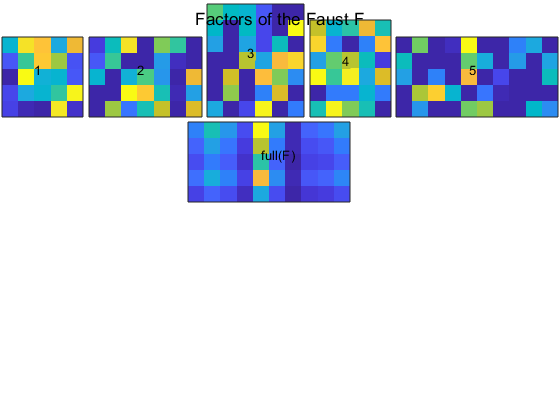What do we see above? On the bottom is the dense matrix associated to F, obtained with full(F). On the top are the indexed factors of F. Note that you can change the default colormap.
Let's look at a last example:
imagesc(Faust({eye(5,4),eye(4,10)}))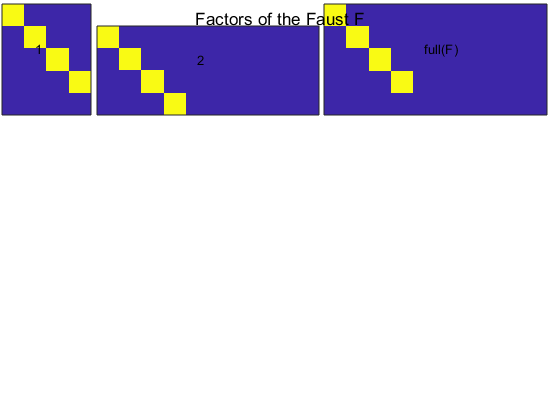Three functions of the Faust class are here to evaluate the sparsity of a Faust object.
Let's call the first one:
nnz_sum(F)
ans = 133
I'm sure you guessed exactly what the function returns, if you doubt it, here is the doc: Faust.nnz_sum(). The smaller nnz_sum, the sparser the Faust.
Next comes the function: Faust.density().
This function along with its reciprocal Faust.rcg() can give you a big hint on how much your Faust is potentially optimized both for storage and calculation. The sparser the Faust, the larger the Relative Complexity Gain (RCG)!
density(F)
ans = 2.6600
rcg(F)
ans = 0.3759
According to its RCG, this Faust doesn't seem to be of any help for optimization but look at the graphic the next script generates:
figure()
nfactors = 3;
startd = 0.01;
endd = 1;
dim_sz = 1000;
ntests = 10;
sizes = zeros(ntests, 1);
rcs = zeros(ntests, 1);
densities = linspace(startd, endd, ntests);
for i=1:ntests
d = densities(i);
F = matfaust.rand(dim_sz, dim_sz, 'num_factors', nfactors, 'density', d, 'fac_type', 'sparse');
sizes(i) = nbytes(F);
rcs(i) = density(F);
end
plot(rcs, sizes)
legend('size')
xlabel('Density')
ylabel('Faust Size (bytes)')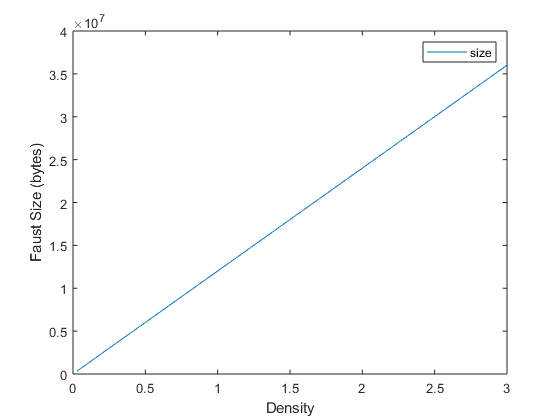Isn't it obvious now that the smaller the density the better?! Indeed, for two Faust objects of the same shape and the same number of factors, a smaller density (linearly) implies a smaller file size for storage. This point applies also to the memory (RAM) space to work on a Faust.
We'll see later how the computation can benefit of a larger RCG (or smaller density). But let's point out right now that when it comes to matrix factorizations the sparsity is often a tradeoff with accuracy, as the following plot shows about the truncated SVD of a matrix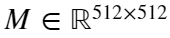. Note beforehand that the SVD of M (truncated or not) can be seen as a Faust which approximates M.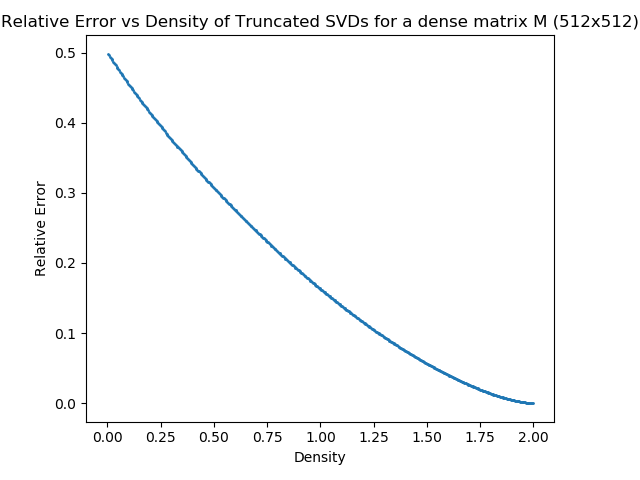Here is the script to reproduce the last figure with pyfaust: test_svd_rc_vs_err.py

### 2. Faust Algebra and other Operations

In order to write some nice algorithms using Faust objects, you'll have to use the basic "stable" operations a Faust is capable of. Let's make a tour of them.

#### 2.1 Transpose, conjugate, transconjugate

You are probably familiar with .' and ' shorthand operators from Matlab. Well, they are also used in the Faust class.
G = matfaust.rand(10, 15, 'dim_sizes', [10,15], 'field', 'complex')
G =
Faust size 10x15, density 2.13333, nnz_sum 320, 5 factor(s): - FACTOR 0 (complex) SPARSE, size 10x11, density 0.454545, nnz 50 - FACTOR 1 (complex) SPARSE, size 11x13, density 0.384615, nnz 55 - FACTOR 2 (complex) SPARSE, size 13x15, density 0.333333, nnz 65 - FACTOR 3 (complex) SPARSE, size 15x15, density 0.333333, nnz 75 - FACTOR 4 (complex) SPARSE, size 15x15, density 0.333333, nnz 75
G.'
ans =
Faust size 15x10, density 2.13333, nnz_sum 320, 5 factor(s): - FACTOR 0 (complex) SPARSE, size 15x15, density 0.333333, nnz 75 - FACTOR 1 (complex) SPARSE, size 15x15, density 0.333333, nnz 75 - FACTOR 2 (complex) SPARSE, size 15x13, density 0.333333, nnz 65 - FACTOR 3 (complex) SPARSE, size 13x11, density 0.384615, nnz 55 - FACTOR 4 (complex) SPARSE, size 11x10, density 0.454545, nnz 50
conj(G)
F_conj =
Faust size 10x15, density 2.13333, nnz_sum 320, 5 factor(s): - FACTOR 0 (complex) SPARSE, size 10x11, density 0.454545, nnz 50 - FACTOR 1 (complex) SPARSE, size 11x13, density 0.384615, nnz 55 - FACTOR 2 (complex) SPARSE, size 13x15, density 0.333333, nnz 65 - FACTOR 3 (complex) SPARSE, size 15x15, density 0.333333, nnz 75 - FACTOR 4 (complex) SPARSE, size 15x15, density 0.333333, nnz 75
ans =
Faust size 10x15, density 2.13333, nnz_sum 320, 5 factor(s): - FACTOR 0 (complex) SPARSE, size 10x11, density 0.454545, nnz 50 - FACTOR 1 (complex) SPARSE, size 11x13, density 0.384615, nnz 55 - FACTOR 2 (complex) SPARSE, size 13x15, density 0.333333, nnz 65 - FACTOR 3 (complex) SPARSE, size 15x15, density 0.333333, nnz 75 - FACTOR 4 (complex) SPARSE, size 15x15, density 0.333333, nnz 75
G'
ans =
Faust size 15x10, density 2.13333, nnz_sum 320, 5 factor(s): - FACTOR 0 (complex) SPARSE, size 15x15, density 0.333333, nnz 75 - FACTOR 1 (complex) SPARSE, size 15x15, density 0.333333, nnz 75 - FACTOR 2 (complex) SPARSE, size 15x13, density 0.333333, nnz 65 - FACTOR 3 (complex) SPARSE, size 13x11, density 0.384615, nnz 55 - FACTOR 4 (complex) SPARSE, size 11x10, density 0.454545, nnz 50
What really matters here is that the results of G.', conj(G) and G' are all Faust objects. Behind the scene, there is just one memory zone allocated to the factors. Strictly speaking they are memory views shared between G, G.' and G'. So don't hesitate to use!

#### 2.2 Add, Subtract and Multiply

s = size(G, 1);
F = matfaust.rand(s, s);
G = matfaust.rand(s, s, 'field', 'complex');
F+G
ans =
Faust size 10x10, density 5.6, nnz_sum 560, 8 factor(s): - FACTOR 0 (complex) SPARSE, size 10x20, density 0.1, nnz 20 - FACTOR 1 (complex) SPARSE, size 20x20, density 0.25, nnz 100 - FACTOR 2 (complex) SPARSE, size 20x20, density 0.25, nnz 100 - FACTOR 3 (complex) SPARSE, size 20x20, density 0.25, nnz 100 - FACTOR 4 (complex) SPARSE, size 20x20, density 0.25, nnz 100 - FACTOR 5 (complex) SPARSE, size 20x20, density 0.25, nnz 100 - FACTOR 6 (complex) SPARSE, size 20x20, density 0.05, nnz 20 - FACTOR 7 (complex) SPARSE, size 20x10, density 0.1, nnz 20
Go ahead and verify it's accurate.
norm(full(F+G)-(full(F)+full(G)), 'fro')
ans = 0
Some points are noticeable here:
• F is real but G is complex. The Faust API is able to return the proper type for the resulting Faust, that is a complex Faust.
• F+G is composed of 8 factors, however F and G are both 5 factors long. It's due to the way the addition is implemented (Faust concatenation is hiding behind).
Subtracting is not different:
F-G
ans =
Faust size 10x10, density 5.6, nnz_sum 560, 8 factor(s): - FACTOR 0 (complex) SPARSE, size 10x20, density 0.1, nnz 20 - FACTOR 1 (complex) SPARSE, size 20x20, density 0.25, nnz 100 - FACTOR 2 (complex) SPARSE, size 20x20, density 0.25, nnz 100 - FACTOR 3 (complex) SPARSE, size 20x20, density 0.25, nnz 100 - FACTOR 4 (complex) SPARSE, size 20x20, density 0.25, nnz 100 - FACTOR 5 (complex) SPARSE, size 20x20, density 0.25, nnz 100 - FACTOR 6 (complex) SPARSE, size 20x20, density 0.05, nnz 20 - FACTOR 7 (complex) SPARSE, size 20x10, density 0.1, nnz 20
You can also add/subtract scalars to Faust objects.
F+2
ans =
Faust size 10x10, density 3.6, nnz_sum 360, 8 factor(s): - FACTOR 0 (double) SPARSE, size 10x20, density 0.1, nnz 20 - FACTOR 1 (double) SPARSE, size 20x20, density 0.15, nnz 60 - FACTOR 2 (double) SPARSE, size 20x20, density 0.15, nnz 60 - FACTOR 3 (double) SPARSE, size 20x20, density 0.15, nnz 60 - FACTOR 4 (double) SPARSE, size 20x11, density 0.272727, nnz 60 - FACTOR 5 (double) SPARSE, size 11x20, density 0.272727, nnz 60 - FACTOR 6 (double) SPARSE, size 20x20, density 0.05, nnz 20 - FACTOR 7 (double) SPARSE, size 20x10, density 0.1, nnz 20
Note that here again numfactors(F+2) ~= numfactors(F).
The FAµST API supports equally the Faust to array addition and subtraction.
F+full(F)
ans =
Faust size 10x10, density 4.5, nnz_sum 450, 8 factor(s): - FACTOR 0 (double) SPARSE, size 10x20, density 0.1, nnz 20 - FACTOR 1 (double) SPARSE, size 20x20, density 0.15, nnz 60 - FACTOR 2 (double) SPARSE, size 20x20, density 0.15, nnz 60 - FACTOR 3 (double) SPARSE, size 20x20, density 0.15, nnz 60 - FACTOR 4 (double) SPARSE, size 20x20, density 0.15, nnz 60 - FACTOR 5 (double) SPARSE, size 20x20, density 0.375, nnz 150 - FACTOR 6 (double) SPARSE, size 20x20, density 0.05, nnz 20 - FACTOR 7 (double) SPARSE, size 20x10, density 0.1, nnz 20
F-full(F)
ans =
Faust size 10x10, density 4.5, nnz_sum 450, 8 factor(s): - FACTOR 0 (double) SPARSE, size 10x20, density 0.1, nnz 20 - FACTOR 1 (double) SPARSE, size 20x20, density 0.15, nnz 60 - FACTOR 2 (double) SPARSE, size 20x20, density 0.15, nnz 60 - FACTOR 3 (double) SPARSE, size 20x20, density 0.15, nnz 60 - FACTOR 4 (double) SPARSE, size 20x20, density 0.15, nnz 60 - FACTOR 5 (double) SPARSE, size 20x20, density 0.375, nnz 150 - FACTOR 6 (double) SPARSE, size 20x20, density 0.05, nnz 20 - FACTOR 7 (double) SPARSE, size 20x10, density 0.1, nnz 20
all(all(full(F-full(F)) < eps))
ans = logical
1
Now let's multiply these Fausts!
FG = F*G
FG =
Faust size 10x10, density 5, nnz_sum 500, 10 factor(s): - FACTOR 0 (complex) SPARSE, size 10x10, density 0.5, nnz 50 - FACTOR 1 (complex) SPARSE, size 10x10, density 0.5, nnz 50 - FACTOR 2 (complex) SPARSE, size 10x10, density 0.5, nnz 50 - FACTOR 3 (complex) SPARSE, size 10x10, density 0.5, nnz 50 - FACTOR 4 (complex) SPARSE, size 10x10, density 0.5, nnz 50 - FACTOR 5 (complex) SPARSE, size 10x10, density 0.5, nnz 50 - FACTOR 6 (complex) SPARSE, size 10x10, density 0.5, nnz 50 - FACTOR 7 (complex) SPARSE, size 10x10, density 0.5, nnz 50 - FACTOR 8 (complex) SPARSE, size 10x10, density 0.5, nnz 50 - FACTOR 9 (complex) SPARSE, size 10x10, density 0.5, nnz 50
norm(full(FG)-full(F)*full(G))/norm(full(F)*full(G))
ans = 9.9308e-17
Faust scalar multiplication is also available and here again the result is a Faust object!
F*2
ans =
Faust size 10x10, density 2.5, nnz_sum 250, 5 factor(s): - FACTOR 0 (double) SPARSE, size 10x10, density 0.5, nnz 50 - FACTOR 1 (double) SPARSE, size 10x10, density 0.5, nnz 50 - FACTOR 2 (double) SPARSE, size 10x10, density 0.5, nnz 50 - FACTOR 3 (double) SPARSE, size 10x10, density 0.5, nnz 50 - FACTOR 4 (double) SPARSE, size 10x10, density 0.5, nnz 50

#### 2.3 Faust Multiplication by a Vector or a Matrix

When you multiply a Faust by a vector or a matrix (the number of rows must match the number of Faust columns), you'll get respectively a vector or a matrix as result.
vec = rand(size(F, 2), 1);
F*vec
ans = 10×1
31.6836 39.9588 31.8965 48.1275 46.7694 42.3895 39.7068 57.2432 44.3258 34.7391
Let's launch a timer to compare the execution times of Faust-vector multiplication and Faust's dense matrix-vector multiplication.
F_times_vec = @() F*vec
F_times_vec = function_handle with value:
@()F*vec
FD = full(F);
FD_times_vec = @() FD*vec
FD_times_vec = function_handle with value:
@()FD*vec
timeit(F_times_vec)
ans = 0.0034
timeit(FD_times_vec)
Warning: The measured time for F may be inaccurate because it is running too fast. Try measuring something that takes longer.
ans = 1.1135e-06
all(all(F*vec-FD*vec < 1e-7))
ans = logical
1
rcg(F)
ans = 0.4000
When the RCG is lower than 1 the Faust-vector multiplication is slower. Making a random Faust with a large RCG (small density) shows better results.
G = matfaust.rand(4096, 4096, 'num_factors', 2, 'density', .001, 'fac_type', 'sparse')
G =
Faust size 4096x4096, density 0.00195313, nnz_sum 32768, 2 factor(s): - FACTOR 0 (double) SPARSE, size 4096x4096, density 0.000976563, nnz 16384 - FACTOR 1 (double) SPARSE, size 4096x4096, density 0.000976563, nnz 16384
GD = full(G);
vec2 = rand(size(G, 2), 1);
timeit(@() G*vec2)
ans = 0.0034
timeit(@() GD*vec2)
ans = 0.0231
rcg(G)
ans = 512
It goes without saying that a big RCG gives a big speedup to the Faust-vector multiplication relatively to the corresponding (dense) matrix-vector multiplication. I hope the example above has finished to convince you.
Just to convince you as well of the Faust-vector multiplication accuracy:
norm(G*vec2 - GD*vec2)
ans = 2.0596e-14
What applies to Faust-vector multiplication remains valid about Faust-matrix multiplication. Take a look:
M = rand(size(G, 2), 1024);
timeit(@() G*M)
ans = 0.6241
timeit(@() GD*M)
ans = 2.5308
norm(GD*M-G*M)/norm(GD*M)
ans = 1.5585e-17
Well, what do we see? A quicker Faust-matrix multiplication than the matrix-matrix corresponding multiplication, though a good accuracy of the Faust-matrix multiplication is also clearly confirmed.
These examples are somehow theoretical because we cherry-pick the Faust to ensure that the RCG is good to accelerate the muplication, but at least it shows the potential speedup using Faust objects.

#### 2.4 Faust Norms

The Faust class provides a norm function which handles different types of norms. This function is really close to Matlab norm function.
In the following example, three of the four norms available are computed.
norm(F,1)
ans = 190.5393
norm(F, inf)
ans = 154.7970
norm(F, 'fro')
ans = 124.7755
norm(full(F), 1)
ans = 190.5393
norm(full(F), inf)
ans = 154.7970
norm(full(F), 'fro')
ans = 124.7755
Perfect! But a last norm is available, this is the Faust's 2-norm. Let's see in the next small benchmark how the Faust 2-norm is being computed faster than the Faust's dense matrix 2-norm.
timeit(@() norm(G, 2))
ans = 0.0106
timeit(@() norm(GD, 2))
ans = 83.1448
The power-iteration algorithm implemented in the FAµST C++ core is faster on G and the relative error is not bad too. The norm computation is faster as it benefits from faster Faust-vector multiplication.
err = abs((norm(G, 2)-norm(GD,2))/norm(GD,2))
err = 1.7019e-06

#### 2.5 Faust Normalizations

The FAµST API proposes a group of normalizations. They correspond to the norms available and discussed above.
It's possible to normalize along columns or rows with any type of these norms.
F = matfaust.rand(5, 10)
F =
Faust size 5x10, density 3.9, nnz_sum 195, 5 factor(s): - FACTOR 0 (double) SPARSE, size 5x10, density 0.5, nnz 25 - FACTOR 1 (double) SPARSE, size 10x5, density 1, nnz 50 - FACTOR 2 (double) SPARSE, size 5x10, density 0.5, nnz 25 - FACTOR 3 (double) SPARSE, size 10x9, density 0.555556, nnz 50 - FACTOR 4 (double) SPARSE, size 9x10, density 0.5, nnz 45
NF = normalize(F)
Faust size 5x10, density 3.9, nnz_sum 195, 5 factor(s): - FACTOR 0 (double) SPARSE, size 5x10, density 0.5, nnz 25 - FACTOR 1 (double) SPARSE, size 10x5, density 1, nnz 50 - FACTOR 2 (double) SPARSE, size 5x10, density 0.5, nnz 25 - FACTOR 3 (double) SPARSE, size 10x9, density 0.555556, nnz 50 - FACTOR 4 (double) SPARSE, size 9x10, density 0.5, nnz 45 Faust size 5x10, density 3.9, nnz_sum 195, 5 factor(s): - FACTOR 0 (double) SPARSE, size 5x10, density 0.5, nnz 25 - FACTOR 1 (double) SPARSE, size 10x5, density 1, nnz 50 - FACTOR 2 (double) SPARSE, size 5x10, density 0.5, nnz 25 - FACTOR 3 (double) SPARSE, size 10x9, density 0.555556, nnz 50 - FACTOR 4 (double) SPARSE, size 9x10, density 0.5, nnz 45
NF =
Faust size 5x10, density 3.9, nnz_sum 195, 5 factor(s): - FACTOR 0 (double) SPARSE, size 5x10, density 0.5, nnz 25 - FACTOR 1 (double) SPARSE, size 10x5, density 1, nnz 50 - FACTOR 2 (double) SPARSE, size 5x10, density 0.5, nnz 25 - FACTOR 3 (double) SPARSE, size 10x9, density 0.555556, nnz 50 - FACTOR 4 (double) SPARSE, size 9x10, density 0.5, nnz 45
The API doc is here.
What's interesting here is the fact that Faust.normalize returns a Faust object. Combined with slicing (that we will see soon), normalize is useful to write algorithms such as Orthonormal Matching Pursuit (OMP), which require matrices with L2-normalized columns, in a way that makes them able to leverage the acceleration offered by the FAµST API.
The normalization coded in C++ is memory optimized (it never builds the dense matrix full(F) to compute the norms of the columns/rows). In the same goal the factors composing the Faust object NF are not duplicated in memory from same factors F, they're used as is with an additional factor giving the appropriate scaling.
factors(NF, 5)
ans =
(2,1) 0.0365 (3,1) 0.0111 (6,1) 0.0259 (8,1) 0.0455 (1,2) 0.0245 (2,2) 0.0378 (4,2) 0.0232 (6,2) 0.0427 (9,2) 0.0046 (2,3) 0.0068 (4,3) 0.0439 (5,3) 0.0162 (6,3) 0.0429 (7,3) 0.0478 (1,4) 0.0305 (4,4) 0.0064 (5,4) 0.0249 (7,4) 0.0034 (9,4) 0.0398 (2,5) 0.0209 (5,5) 0.0636 (8,5) 0.0366 (1,6) 0.0368 (3,6) 0.0344 (8,6) 0.0226 (1,7) 0.0121 (4,7) 0.0323 (6,7) 0.0291 (7,7) 0.0197 (9,7) 0.0302 (3,8) 0.0232 (4,8) 0.0301 (5,8) 0.0060 (8,8) 0.0175 (9,8) 0.0196 (1,9) 0.0129 (3,9) 0.0492 (5,9) 0.0176 (7,9) 0.0608 (2,10) 0.0010 (3,10) 0.0654 (6,10) 0.0295 (7,10) 0.0232 (8,10) 0.0065 (9,10) 0.0005
cumerr = 0;
fullF = full(F);
for i=1:size(F,2)
normalized_col = fullF(:,i)/norm(fullF(:,i));
cumerr = cumerr + norm(NF(:,i) - normalized_col, 'fro');
end
cumerr
cumerr = 1.4034e-15
And as you see it works!

#### 2.6 Faust Concatenation

You're probably aware of Matlab arrays concatenation otherwise look this example.
M = rand(5,5);
I = eye(5,5);
[ M; I ]
ans = 10×5
0.7706 0.3175 0.3668 0.7896 0.7740 0.3435 0.0740 0.3750 0.7270 0.4487 0.3992 0.7456 0.3487 0.7266 0.8804 0.9791 0.9123 0.7792 0.8482 0.2689 0.1250 0.4198 0.9776 0.1534 0.0244 1.0000 0 0 0 0 0 1.0000 0 0 0 0 0 1.0000 0 0 0 0 0 1.0000 0 0 0 0 0 1.0000
% it was vertical concatenation, now let's concatenate horizontally
[ M I ]
ans = 5×10
0.7706 0.3175 0.3668 0.7896 0.7740 1.0000 0 0 0 0 0.3435 0.0740 0.3750 0.7270 0.4487 0 1.0000 0 0 0 0.3992 0.7456 0.3487 0.7266 0.8804 0 0 1.0000 0 0 0.9791 0.9123 0.7792 0.8482 0.2689 0 0 0 1.0000 0 0.1250 0.4198 0.9776 0.1534 0.0244 0 0 0 0 1.0000
I'm sure you guessed that likewise you can concatenate Faust objects. That's right!
[ F ; F]
ans =
Faust size 10x10, density 4.1, nnz_sum 410, 6 factor(s): - FACTOR 0 (double) SPARSE, size 10x20, density 0.25, nnz 50 - FACTOR 1 (double) SPARSE, size 20x10, density 0.5, nnz 100 - FACTOR 2 (double) SPARSE, size 10x20, density 0.25, nnz 50 - FACTOR 3 (double) SPARSE, size 20x18, density 0.277778, nnz 100 - FACTOR 4 (double) SPARSE, size 18x20, density 0.25, nnz 90 - FACTOR 5 (double) SPARSE, size 20x10, density 0.1, nnz 20
C = [ F F ]
C =
Faust size 5x20, density 4, nnz_sum 400, 6 factor(s): - FACTOR 0 (double) SPARSE, size 5x10, density 0.2, nnz 10 - FACTOR 1 (double) SPARSE, size 10x20, density 0.25, nnz 50 - FACTOR 2 (double) SPARSE, size 20x10, density 0.5, nnz 100 - FACTOR 3 (double) SPARSE, size 10x20, density 0.25, nnz 50 - FACTOR 4 (double) SPARSE, size 20x18, density 0.277778, nnz 100 - FACTOR 5 (double) SPARSE, size 18x20, density 0.25, nnz 90
full(C) - [ full(F) full(F) ]
ans = 5×20
0 0 0 0 0 0 0 0 0 0 0 0 0 0 0 0 0 0 0 0 0 0 0 0 0 0 0 0 0 0 0 0 0 0 0 0 0 0 0 0 0 0 0 0 0 0 0 0 0 0 0 0 0 0 0 0 0 0 0 0 0 0 0 0 0 0 0 0 0 0 0 0 0 0 0 0 0 0 0 0 0 0 0 0 0 0 0 0 0 0 0 0 0 0 0 0 0 0 0 0
The difference of the two concatenations is full of zeros, so of course it works!
As you noticed the Faust concatenation is stable, you give two Fausts and you get a Faust again. Besides, it's possible to concatenate an arbitrary number of Faust objects.
[F C C F ]
ans =
Faust size 5x60, density 4.15, nnz_sum 1245, 9 factor(s): - FACTOR 0 (double) SPARSE, size 5x10, density 0.2, nnz 10 - FACTOR 1 (double) SPARSE, size 10x15, density 0.1, nnz 15 - FACTOR 2 (double) SPARSE, size 15x20, density 0.0666667, nnz 20 - FACTOR 3 (double) SPARSE, size 20x30, density 0.05, nnz 30 - FACTOR 4 (double) SPARSE, size 30x60, density 0.0833333, nnz 150 - FACTOR 5 (double) SPARSE, size 60x30, density 0.166667, nnz 300 - FACTOR 6 (double) SPARSE, size 30x60, density 0.0833333, nnz 150 - FACTOR 7 (double) SPARSE, size 60x54, density 0.0925926, nnz 300 - FACTOR 8 (double) SPARSE, size 54x60, density 0.0833333, nnz 270
As an exercise, you can write the factors of the Faust [F ; F], F being any Faust.
Hint: the block-diagonal matrices are around here.

#### 2.7 Faust Indexing and Slicing

Sometimes you need to access the dense matrix corresponding to a Faust or an element of it (by the way, note that it's costly).
Let's access a Faust item:
F(3, 4)
ans = 6.1501
Why is it costly? Because it essentially converts the Faust to its dense form (modulo some optimizations) to just access one item.
timeit(@() F(3, 4))
ans = 0.0040
timeit(@() FD(3, 4))
Warning: The measured time for F may be inaccurate because it is running too fast. Try measuring something that takes longer.
ans = 0
It's totally the same syntax as Matlab but much slower so use it with care.
The more advanced slicing operation uses also the same syntax as Matlab:
F(3:5, 4:10)
ans =
Faust size 3x7, density 8.14286, nnz_sum 171, 5 factor(s): - FACTOR 0 (double) SPARSE, size 3x10, density 0.5, nnz 15 - FACTOR 1 (double) SPARSE, size 10x5, density 1, nnz 50 - FACTOR 2 (double) SPARSE, size 5x10, density 0.5, nnz 25 - FACTOR 3 (double) SPARSE, size 10x9, density 0.555556, nnz 50 - FACTOR 4 (double) SPARSE, size 9x7, density 0.492063, nnz 31
Here again, the result is another Faust. But this is not a full copy, it makes profit of memory views implemented behind in C++. Solely the first and last factors of the sliced Faust are new in memory, the others are just referenced from the initial Faust F. So use it with no worry for a Faust with a lot of factors!
The Matlab indexing by an arbitrary vector of integers has also been implemented in the FAµST C++ core, let's try it:
I = [2, 4, 3];
FI = F(I,:);
matfaust.isFaust(FI)
ans = logical
1
Again, it's a Faust but is it really working? Verify!
FID = full(FI)
FID = 3×10
10.0557 10.0557 11.5732 10.0557 10.5396 10.0557 8.3874 10.0557 7.3311 10.0557 12.1712 12.1712 13.8729 12.1712 12.7075 12.1712 9.6538 12.1712 9.3219 12.1712 3.9160 3.9160 4.5326 3.9160 4.2798 3.9160 3.2294 3.9160 2.8998 3.9160
FD = full(F)
FD = 5×10
12.1712 13.8729 12.7075 9.6538 9.3219 14.1815 17.3174 18.3561 7.4647 7.9888 10.0557 11.5732 10.5396 8.3874 7.3311 12.2536 15.1256 15.9729 6.5114 7.1592 7.6369 8.7283 7.7635 6.1501 5.8475 8.9170 10.8106 11.4893 4.6974 5.1123 3.9160 4.5326 4.2798 3.2294 2.8998 4.7012 5.9944 6.3855 2.5417 2.8407 7.4951 8.6031 7.6319 6.0946 5.6265 8.8779 10.7166 11.2073 4.6522 4.9810
all(all(FID(1, :) == FD(I(1), :) & FID(2, :) == FD(I(2), :) & FID(3, :) == FD(I(3), :)))
ans = logical
0
Yes it is!
This is the livescript end, you have now a global view of what the Faust class is able and what kind of high-level algorithms it is ready for. You might be interested to read other livescripts, just go back to the page where you got this one.
Note: this livescript was executed using the following matfaust version:
matfaust.version()
ans = '3.27.2'